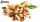# Eq-frac

Solve the following equation with fractions:

h + 1/3 =5/3

Result

h =  1.333

#### Solution:

h + 1/3 =5/3

3h = 4

h = 43 ≈ 1.333333

Calculated by our simple equation calculator.

Leave us a comment of example and its solution (i.e. if it is still somewhat unclear...):Be the first to comment!#### To solve this example are needed these knowledge from mathematics:

Need help calculate sum, simplify or multiply fractions? Try our fraction calculator. Do you have a linear equation or system of equations and looking for its solution? Or do you have quadratic equation?

## Next similar examples:

1. Equation with xSolve the following equation: 2x- (8x + 1) - (x + 2) / 5 = 9
2. EqnSolve equation with fractions: 2x/3-50=40+x/4
3. EquationSolve equation and check the result: 1.4x - 3/2 + x - 9,8 = x + 0,4/3 - 7 + 1,6/6
4. Simple equationSolve for x: 3(x + 2) = x - 18
5. Unknown numberI think the number - its sixth is 3 smaller than its third.
6. PearsThere were pears in the basket, I took two-fifths of them, and left six in the basket. How many pears did I take?
7. One thirdIf 3/5 is 360, how much is 1/3?
8. NumberI think the number. If I add to its third seven I get same as when to its quarter add 8. Which is the number?
9. Cleaning windowsCleaning company has to wash all the windows of the school. The first day washes one-sixth of the windows of the school, the next day three more windows than the first day and the remaining 18 windows washes on the third day. Calculate how many windows ha
10. Missing numberBlank +1/6 =3/2 find the missing number
11. UN 1If we add to an unknown number his quarter, we get 210. Identify unknown number.
12. TreesFrom the total number of trees in the orchard, there are two-fifths pearls and apples are three eighty. The rest of the trees are 9 ceremonial. How many trees are in the set?
13. EquationFind x: x + 1/2 = 1/3
14. Bag of peanutsJoe eat 1/3 of a bag of peanuts, mark eat 1/4 of the remaining in the bag of peanuts, Alvin eat 1/2 of the remaining bag of peanuts, peter eat 10 peanuts, there are 71 peanuts left. Hon many peanuts were in the bags?
15. Grandmother and grandfatherGrandmother baked cakes. Grandfather ate half, then quarter of the rest ate Peter and Paul ate half of rest. For parents left 6 cakes. How many cakes maked the grandmother?
16. The bunsKate, Zofia and Peter Liked buns. Even today, their grandmother prepare their favorite meal. Katka eats 4 bunches, Žofia 3 and Petra eats 5 buns. Their grandmother said to them, "My inmate will you know how many buns I have been make today, if those you ea
17. Unknown number 6Determine x if 1/6 of x is equal to 2/5 of the number 24.